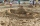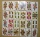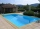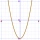# Algebra - problems - page 103

1. Sand castleTim and Tom built a sand castle and embellished it with a flag. Half the pole with the flag plunged into the castle. The highest point of the pole was 80 cm above the ground, its lowest point 20 cm above the ground. How high was the sand castle?
2. Geometric progression 48,4√2,4,2√2
3. Rectangular trapezoidIn a rectangular trapezoid ABCD with right angles at vertices A and D with sides a = 12cm, b = 13cm, c = 7cm. Find the angles beta and gamma and height v.
4. EquatiomSolve equation with negatives: X/(-5) + 2 = -9
5. AlgebraX+y=5, find xy (find the product of x and y if x+y = 5)
6. Mba studiumAt MBA school, fourth-year students can choose from three optional subjects: a) mathematical methods, b) social interaction, c) management Each student studies one of these subjects. The mathematical methods studied 28 students, the social interaction 27.
7. MultiplesWhat is the sum of the multiples of number 7 that are greater than 30 but less than 56?
8. LegsCancer has 5 pairs of legs. The insect has 6 legs. 60 animals have a total of 500 legs. How much more are cancers than insects?
9. In a 2In a thirteen sided polygon, the sum of five angles is 1274°, four of the eight angles remaining are equal and the other four are 18° less than each of the equal angles. Find the angles. .
10. EmployeesThe company employs 1 440 employees (men and women). For over-average results, the premiums were 18.75% of all men and 22.5% of all women. 20% of employees were rewarded with premiums. How many men and how many women are employed in the company?
11. Ratio of volumesIf the heights of two cylindrical drums are in the ratio 7:8 and their base radii are in the ratio 4:3. What is the ratio of their volumes?
12. Three tributariesIt is possible to fill the pool with three tributaries. The first would take 12 hours, the second 15 hours, and the third 20 hours. The day before the summer season began, the manager opened all three tributaries simultaneously. How long did it take to fil
13. Diofant equation250x + 120y = 5640
14. A triangleA triangle has an angle that is 63.1 other 2 are in ratio of 2:5 What are the measurements of the two angles?
15. Calculate 3Calculate the cube volume whose edge is 3x-1,3x-1,3x-1
16. Domains of functionsF(x)=x2-7x and g(x)=5-x2 Domain of (fg)(x) is. .. . . Domain of (f/g)(x). ..
17. A rectangleA rectangle has an area of 36 cm2. What could the length and width of rectangle be?
18. Midpoint 4If the midpoint of a segment is (6,3) and the other end point is (8,-4) what are thw coordinate of the other end?
19. Two unknown numbersOne number is 2 less than a second number. Twice the second number is 14 less than 5 times the first. Find the two numbers.
20. Linear systemSolve a set of two equations of two unknowns: 1.5x+1.2y=0.6 0.8x-0.2y=2

Do you have an interesting mathematical problem that you can't solve it? Enter it, and we can try to solve it.

To this e-mail address, we will reply solution; solved examples are also published here. Please enter e-mail correctly and check whether you don't have a full mailbox.